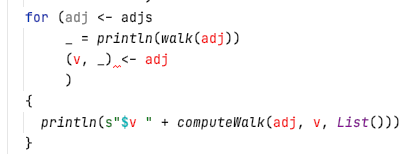# Do I need a semicolon in a for comprehension?

Are more semicolons required in 2.13 than were needed in 2.12, particularly in `for` comprehensions?

The following function works as I expect. Both IntelliJ and the scala compiler are happy.

``````  def main(argv: Array[String]): Unit = {
val adjs = Seq(Map(1 -> Set(2, 3),
2 -> Set(1, 4),
3 -> Set(1, 4),
4 -> Set(2, 3, 5, 6, 7, 8),
5 -> Set(4, 6),
6 -> Set(4, 5),
7 -> Set(4, 8),
8 -> Set(4, 7)),
Map(1 -> Set(2),
2 -> Set(1))
)
)
{
println(s"\$v " + computeWalk(adj, v, List()))
}
}
``````

However, if I remove the semicolons, I get compilation errors.

``````  def main(argv: Array[String]): Unit = {
val adjs = Seq(Map(1 -> Set(2, 3),
2 -> Set(1, 4),
3 -> Set(1, 4),
4 -> Set(2, 3, 5, 6, 7, 8),
5 -> Set(4, 6),
6 -> Set(4, 5),
7 -> Set(4, 8),
8 -> Set(4, 7)),
Map(1 -> Set(2),
2 -> Set(1))
)
_ = println(walk(adj)) // no semicolon
)
{
println(s"\$v " + computeWalk(adj, v, List()))
}
}
``````

Here are the errors:

``````/Users/jimka/Repos/algo/tp-theg/scala/theg/src/main/scala/theg/EulerianWalk.scala:106:12
')' expected but '=' found.
/Users/jimka/Repos/algo/tp-theg/scala/theg/src/main/scala/theg/EulerianWalk.scala:107:17
';' expected but '<-' found.
``````

Also IntelliJ highlights the same errors (apparently) as the compiler identifies.You need semicolons if you use parens. If you use braces you can omit semicolons if you separate statements with newlines.

``````for {
_ = println(walk(adj)) // no semicolon
}  {
println(s"\$v " + computeWalk(adj, v, List()))
}
``````

I use parens for the for enumerator list very seldom.

BTW, this is because of Scala semicolon intference rules. In short, a line ending is treated as a semicolon unless one of the following conditions is true:

1. The line in question ends in a word that would not be legal as the end of a statement, such as a period or an infix operator.
2. The next line begins with a word that cannot start a statement.
3. The line ends while inside parentheses(…) or brackets[…], because these cannot contain multiple statements anyway.

(rule 3 is the one relevant here)

Note that this is a rather simplified view. The full rules are in section 1.2 Newline Characters of the Scala Language Specification.

(I don’t think this has changed between Scala versions, has been the case since 2.10 IIRC)

2 Likes

Thanks. yes, when I use braces rather than parens, everyone is happy.